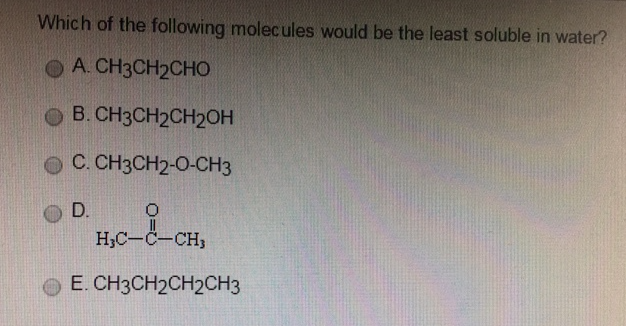# Problem: Which of the following molecules would be the least soluble in water?

###### FREE Expert Solution

We’re being asked to determine the compound that is least soluble in water.

Recall that the main idea in dissolution is like dissolves like, which means compounds with the same polarity and intermolecular force can dissolve each other.

Water is a polar molecule that exhibits hydrogen bonding, so the least soluble compound is a nonolar compound. For this problem, we need to determine the polarity of each molecule.

83% (227 ratings)###### Problem Details

Which of the following molecules would be the least soluble in water?